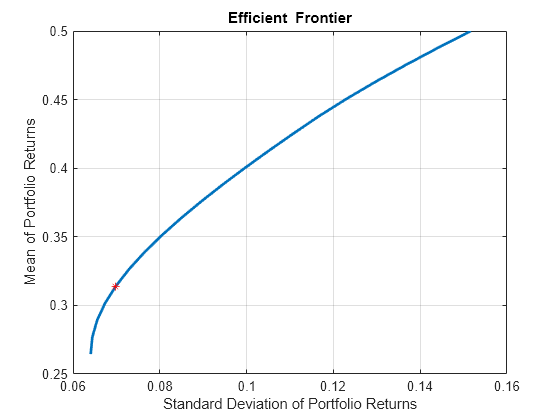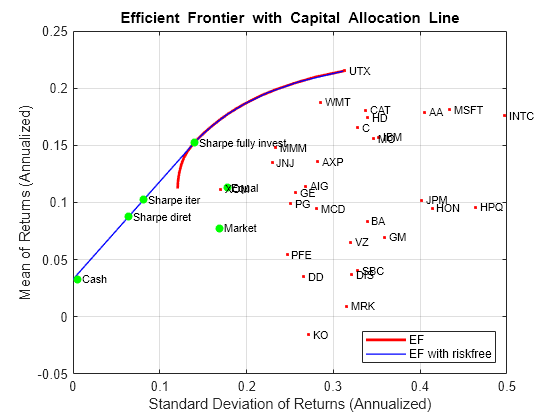# estimateMaxSharpeRatio

## 语法

``[pwgt,pbuy,psell] = estimateMaxSharpeRatio(obj)``
``[pwgt,pbuy,psell] = estimateMaxSharpeRatio(___,Name,Value)``

## 说明

``[pwgt,pbuy,psell] = estimateMaxSharpeRatio(obj)` 估计有效投资组合以最大化 `Portfolio` 对象的夏普比率。有关工作流的详细信息，请参阅 Portfolio 对象工作流。`

``[pwgt,pbuy,psell] = estimateMaxSharpeRatio(___,Name,Value)` 添加了可选的名称-值对组参数。 `

## 示例

```p = Portfolio('AssetMean',[0.3, 0.1, 0.5], 'AssetCovar',... [0.01, -0.010, 0.004; -0.010, 0.040, -0.002; 0.004, -0.002, 0.023]); p = setDefaultConstraints(p); plotFrontier(p, 20); weights = estimateMaxSharpeRatio(p); [risk, ret] = estimatePortMoments(p, weights); hold on plot(risk,ret,'*r');``````p = Portfolio('AssetMean',[0.3, 0.1, 0.5], 'AssetCovar',... [0.01, -0.010, 0.004; -0.010, 0.040, -0.002; 0.004, -0.002, 0.023]); p = setDefaultConstraints(p); plotFrontier(p, 20); p = setSolver(p,'quadprog','Display','off','ConstraintTolerance',1.0e-8,'OptimalityTolerance',1.0e-8,'StepTolerance',1.0e-8,'MaxIterations',10000); weights = estimateMaxSharpeRatio(p); [risk, ret] = estimatePortMoments(p, weights); hold on plot(risk,ret,'*r');``````p = Portfolio('AssetMean',[0.3, 0.1, 0.5], 'AssetCovar',... [0.01, -0.010, 0.004; -0.010, 0.040, -0.002; 0.004, -0.002, 0.023],'lb', 0,'budget', 1); plotFrontier(p, 20); p = setSolver(p, 'fmincon', 'Display', 'off', 'Algorithm', 'sqp', ... 'SpecifyObjectiveGradient', true, 'SpecifyConstraintGradient', true, ... 'ConstraintTolerance', 1.0e-8, 'OptimalityTolerance', 1.0e-8, 'StepTolerance', 1.0e-8); weights = estimateMaxSharpeRatio(p); te = 0.08; p = setTrackingError(p,te,weights); [risk, ret] = estimatePortMoments(p,weights); hold on plot(risk,ret,'*r');````estimateMaxSharpeRatio` 函数可使有效边界上的投资组合中的夏普比率最大化。在具有无风险资产的投资组合的情况下，有多个有效的投资组合可以最大化资产线的夏普比率。鉴于 `'direct'``'iterative'` 方法自身的性质，上述每种方法的投资组合权重 (`pwgts`) 输出可能会有所不同，但夏普比率是相同的。此示例演示了 `pwgts` 不同而夏普比率相同的情形。

```load BlueChipStockMoments mret = MarketMean; mrsk = sqrt(MarketVar); cret = CashMean; crsk = sqrt(CashVar); p = Portfolio('AssetList', AssetList, 'RiskFreeRate', CashMean); p = setAssetMoments(p, AssetMean, AssetCovar); p = setInitPort(p, 1/p.NumAssets); [ersk, eret] = estimatePortMoments(p, p.InitPort); p = setDefaultConstraints(p); pwgt = estimateFrontier(p, 20); [prsk, pret] = estimatePortMoments(p, pwgt); pwgtshpr_fully = estimateMaxSharpeRatio(p,'Method','direct'); [riskshpr_fully, retshpr_fully] = estimatePortMoments(p,pwgtshpr_fully); q = setBudget(p, 0, 1); qwgt = estimateFrontier(q, 20); [qrsk, qret] = estimatePortMoments(q, qwgt);```

```pwgtshpr_direct = estimateMaxSharpeRatio(q,'Method','direct'); pwgtshpr_iter = estimateMaxSharpeRatio(q,'Method','iterative'); % Default for 'TolX' is 1e-8 [riskshpr_diret, retshpr_diret] = estimatePortMoments(q,pwgtshpr_direct); [riskshpr_iter, retshpr_iter] = estimatePortMoments(q,pwgtshpr_iter); clf; portfolioexamples_plot('Efficient Frontier with Capital Allocation Line', ... {'line', prsk, pret, {'EF'}, '-r', 2}, ... {'line', qrsk, qret, {'EF with riskfree'}, '-b', 1}, ... {'scatter', [mrsk, crsk, ersk, riskshpr_fully, riskshpr_diret, riskshpr_iter], ... [mret, cret, eret, retshpr_fully , retshpr_diret, retshpr_iter], {'Market', 'Cash', 'Equal','Sharpe fully invest', 'Sharpe diret','Sharpe iter'}}, ... {'scatter', sqrt(diag(p.AssetCovar)), p.AssetMean, p.AssetList, '.r'}); ``````maxReturn = max(qret); % Max return portfolio minReturn = min(qret); % Min return portfolio display(0.01*(maxReturn-minReturn))```
``` 1.5193e-04 ```

```pwgtshpr_iter_largerTol = estimateMaxSharpeRatio(q, 'Method', 'iterative',... 'TolX', 1e-4); display(table(pwgtshpr_iter, pwgtshpr_iter_largerTol,... 'VariableNames', {'Default TolX = 1e-8','TolX = 1e-4'}))```
``` 30x2 table Default TolX = 1e-8 TolX = 1e-4 ___________________ ___________ 0 0 0 0 0 0 0 0 0 0 0 0 0 0 0 0 0 0 0 0 0 0 0 0 0 0 0 0 0.009173 0.0091742 0.031008 0.031011 0 0 0 0 0 0 0.053382 0.053388 0.048198 0.048204 0 0 0.01542 0.015422 0 0 0.025685 0.025688 0 0 0.020681 0.020684 0 0 0.075935 0.075945 0.064881 0.064889 ```

```AssetMean = [ 0.0101110; 0.0043532; 0.0137058 ]; AssetCovar = [ 0.00324625 0.00022983 0.00420395; 0.00022983 0.00049937 0.00019247; 0.00420395 0.00019247 0.00764097 ]; p = Portfolio('AssetMean', AssetMean, 'AssetCovar', AssetCovar); p = setDefaultConstraints(p); ```

`p = setBounds(p, 0.02, 0.5,'BoundType', 'Conditional', 'NumAssets', 3); `

`p = setMinMaxNumAssets(p, 2, 2); `

`weights = estimateMaxSharpeRatio(p,'Method','iterative')`
```weights = 3×1 0 0.5000 0.5000 ```

`estimateMaxSharpeRatio` 函数使用 MINLP 求解器来求解此问题。使用 `setSolverMINLP` 函数来配置 `SolverType` 和选项。

`p.solverOptionsMINLP`
```ans = struct with fields: MaxIterations: 1000 AbsoluteGapTolerance: 1.0000e-07 RelativeGapTolerance: 1.0000e-05 NonlinearScalingFactor: 1000 ObjectiveScalingFactor: 1000 Display: 'off' CutGeneration: 'basic' MaxIterationsInactiveCut: 30 ActiveCutTolerance: 1.0000e-07 IntMainSolverOptions: [1x1 optim.options.Intlinprog] NumIterationsEarlyIntegerConvergence: 30 ```

## 输入参数

### 名称-值参数

`Name1=Value1,...,NameN=ValueN` 形式指定可选参数对组，其中 `Name` 是参数名称，`Value` 是对应的值。名称-值参数必须显示在其他参数的后面，但参数对的顺序不重要。

## 输出参数

`Portfolio` 输入对象 (`obj`) 返回 `pbuy`

`Portfolio` 输入对象 (`obj`) 返回 `psell`

## 详细信息

### 夏普比率

`estimateMaxSharpeRatio` 函数可使有效边界上的投资组合中的夏普比率最大化。

## 提示

`[pwgt,pbuy,psell] = obj.estimateMaxSharpeRatio;`

## 算法

 Cornuejols, G. and Reha Tütüncü. Optimization Methods in Finance. Cambridge University Press, 2007.